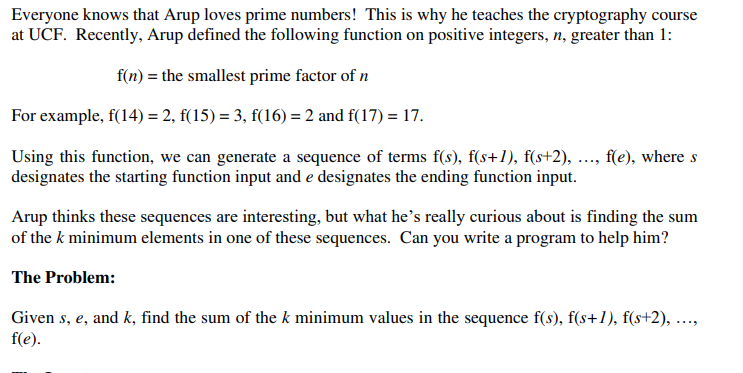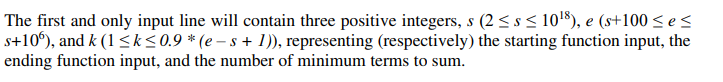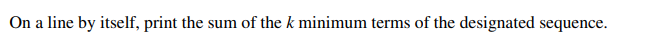# UCF Local Programming Contest Round 1A 训练联盟暑假场1 题解

2021/7/21 12:00:41 浏览：

# UCF Local Programming Contest Round 1A 训练联盟暑假场1 题解

 😊 | Powered By HeartFireY | UCF Local Programming Contest Round 1A Solution

## A.Briefcases Full of Money

 😀 | 知识点：“打字速度”

#include <bits/stdc++.h>
using namespace std;

int a = {0, 1, 5, 10, 20, 50, 100, 200};
int bmax = 0, imax = 0;

signed main(){
for(int i = 1; i <= 6; i++){
int tmp; cin >> tmp;
if(tmp * a[i] >= bmax * a[imax]) imax = i, bmax = tmp;
}
cout << a[imax] << endl;
return 0;
}


## B.A Game Called Mind

 😀 | 知识点：思维

This problem deals with a simplified version of a game called “Mind”. In this game, a group tries to cooperatively solve a problem. Assume:
- There are 2-6 players (called A, B, C, D, E, F).
- Each player has 1-9 cards in sorted order.
- Each card value is between 10 and 99 (inclusive).

- There are no duplicate values, i.e., a card (number) is in at most one hand.

The objective of the game is for all the players to put all the cards down in sorted order (in the real game, players do not see their own cards, hence the name for the game – Mind). Given the cards in each hand, you are to provide the order for the players to place their cards down.

The first input line contains an integer, p (2 ≤ p ≤ 6), indicating the number of players. Each of  the following p input lines provides the cards for one player. Each line starts with an integer, c (1 ≤ c ≤ 9), indicating the number of cards for that player; the count is followed by the cards in sorted order (each card between 10 and 99, inclusive).


Print the order for the players to place down the cards so that the cards are in order.


### 解决方案

#include <bits/stdc++.h>
using namespace std;

struct poke{
int val, player;
bool operator < (struct poke a){ return val < a.val; }
}poke;

char mp[] = {'A', 'B', 'C', 'D', 'E', 'F'};

signed main(){
int t = 0, sum = 0; cin >> t;
for(int i = 0; i < t; i++){
int n = 0; cin >> n;
for(int j = 0; j < n; j++){
cin >> poke[sum].val;
poke[sum++].player = i;
}
}
sort(poke, poke + sum);
for(int i = 0; i < sum; i++)
cout << mp[poke[i].player];
cout << endl;
return 0;
}


## C.Unique Values

 😀 | 知识点：双指针

Arup has to make many practice questions for his Computer Science 1 students. Since many of the questions deal with arrays, he has to generate arrays for his students. Since he doesn’t want to give them difficult practice problems, he always guarantees that the arrays (given to the students) have unique values. Namely, no value will appear twice in any of his arrays.

Unfortunately, Arup is too lazy to generate arrays! About 20 years ago when he started teaching Computer Science 1, he made one really long array to reuse but this long array may have duplicate values. When he makes problems, he simply grabs a contiguous subsequence of this long array to be the array to be used for a problem but he needs to make sure the contiguous subsequence does not contain duplicates. If the long array has terms a, a, …, a[n-1], a contiguous subsequence of the long array is any sequence of j – i + 1 terms a[i], a[i+1], …, a[j] where 0 ≤ i ≤ j ≤ n – 1.

The Problem: Given an array of n integers, determine how many contiguous subsequences of the array do not contain any repeated values. Note that two subsequences with the same contents are considered different (i.e., both counted in the total) if they start at different locations of the original long array.

The first input line contains a single positive integer, n (1 ≤ n ≤ 105 ), representing the size of the input array. The following line contains n space separated integers, representing the values of the input array, in the order they appear in the array. Each of the array values will be an integer between 1 and 109 , inclusive.

On a line by itself, output the total number of contiguous subsequences of the input array that do not contain any repeated values

### 解决方案#include <bits/stdc++.h>
#define ll long long
using namespace std;

const int N = 1e5 + 10;
ll val[N], ans = 0, l = 0;

signed main(){
int n = 0; cin >> n;
for(int i = 0; i < n; i++) cin >> val[i];
set<int> s;
for(int h = 0; h < n; h++){
while(s.count(val[h]) == 1) s.erase(val[l++]);
s.insert(val[h]);
ans += (h - l + 1);
}
cout << ans << endl;
return 0;
}


## D.Gone Fishing

 😀 | 知识点：计算几何基础

WA了5发，全都是因为考虑不周全QAQ。AC CODE:

#include <bits/stdc++.h>
#define mkp make_pair
using namespace std;

const int N = 100 + 10;
const double JD = 0.0000001;

typedef pair<double, double> pdd;

inline double getdis(double x1 ,double y1, double x2, double y2){ return (double)sqrt((x2 - x1) * (x2 - x1) + (y2 - y1) * (y2 - y1)); }

pdd point[N];

inline void getcirclecenter(double x1, double y1, double x2, double y2, double R, double &xx1, double &yy1, double &xx2, double yy2){
if(x2 == x1){
xx1 = x1 + sqrt(R * R - ((y2 - y1) * (y2 - y1) / 4)), yy1 = (y2 + y1) * 0.5, xx2 = x1 - sqrt(R * R - ((y2 - y1) * (y2 - y1) / 4)), yy2 = yy1;
return;
}
double x, y;
double c1 = (x2 * x2 - x1 * x1 + y2 * y2 - y1 * y1) / (2 * (x2 - x1));
double c2 = (y2 - y1) / (x2 - x1);
double A = (c2 * c2 + 1), B = (2 * x1 * c2 - 2 * c1 * c2 - 2 * y1), C = x1 * x1 - 2 * x1 * c1 + c1 * c1 + y1 * y1 - R * R;
yy1 = (-B + sqrt(B * B - 4 * A * C)) / (2 * A);
xx1 = c1 - c2 * yy1;
yy2 = (-B - sqrt(B * B - 4 * A * C)) / (2 * A);
xx2 = c1 - c2 * yy2;
}

signed main(){
double r = 0; cin >> r;
int n = 0; cin >> n;
int ans = 0;
for(int i = 0; i < n; i++) cin >> point[i].first >> point[i].second;
for(int i = 0; i < n; i++){
for(int j = i + 1; j < n; j++){
if(getdis(point[i].first, point[i].second, point[j].first, point[j].second) > 2 * r) continue;
double ncx1, ncy1, ncx2, ncy2;
getcirclecenter(point[i].first, point[i].second, point[j].first, point[j].second, r, ncx1, ncy1, ncx2, ncy2);
int tans1 = 0, tans2 = 0;
for(int k = 0; k < n; k++){
//if(k == i || k == j) continue;
double dis1 = getdis(ncx1, ncy1, point[k].first, point[k].second) - r；
double dis2 = getdis(ncx2, ncy2, point[k].first, point[k].second) - r;
if(dis1 < JD) tans1++;
if(dis2 < JD) tans2++;
}
ans = max(max(tans1, tans2), ans);
}
}
ans = max(ans, 1);
cout << ans;
return 0;
}


## E.Sum of a Function

 😀 | 知识点：素数筛、容斥原理### 题目分析

r r ​的计算：对于区间内的数字，我们采用以下形式进行表示：

#include <bits/stdc++.h>
#define ll long long
using namespace std;

const int MAXN = 1e6 + 1010;

ll prime[MAXN], isp[MAXN], res[MAXN], cnt = 0;

void init(){
for(int i = 2; i < MAXN; i++){
if(!isp[i]) prime[++cnt] = i;
for(int j = 1; j <= cnt && i * prime[j] < MAXN; j++){
isp[prime[j] * i] = 1;
if(i % prime[j] == 0) break;
}
}
}

void solve(ll l, ll r){
for(int i = 0 ; i < r - l + 1; i++) res[i] = i + l;
for(int i = 1; i <= cnt; i++){
ll s = l / prime[i];
if(s <= 1) s = 2;
for(ll j = s * prime[i]; j <= r; j += prime[i]){
if(j >= l){
if(res[j - l] == j) res[j - l] = prime[i];
}
}
}
}

signed main(){
init();
int s, e, k; cin >> s >> e >> k;
solve(s, e);
sort(res, res + e - s + 1);
ll sum = 0;
for(int i = 0; i < k; i++) sum += res[i];
cout << sum << endl;
return 0;
}


## F.Hang Gliding

 😀 | 知识点：DP动态规划

The 2020 hang gliding world championships are coming to Florida next spring! (You may think it is odd to hold in Florida a sport that requires mountains, but it turns out that hang gliders can be towed to altitude by other aircraft.) The competition is divided into a set of tasks; completing a task successfully gives a pilot a number of points. Either all points are awarded for a task, or none are. For each task, every pilot has a probability of success. A pilot’s score is the total of all successfully completed tasks at the end of the competition.

This year, the organizing committee couldn’t decide on a reasonable number of tasks, so the time slots for tasks overlap. At any given time, there can be multiple tasks going on, but a pilot may only choose one to be competing in at that time. Each pilot may compete in as many tasks as they want given this constraint. The pilots know their own strengths and weaknesses, and will choose tasks in order to maximize their expected score.

You have been offered free hang gliding lessons if you help with scoring software for the event. Among other things, the committee wants the software to be able to predict the winners ahead of time. Given a set of tasks, each with a time slot and a point score to be awarded for success, and a list of pilots, each with success probabilities for each task, compute the expected score for each pilot, and report the top 3 expected scores.

The first input line contains two integers: t (1 ≤ t ≤ 10000), indicating the number of tasks, and p(3 ≤ p ≤ 100), indicating the number of pilots.

The next t input lines contain the task descriptions. Each line contains three integers (s, e, and a) separated by a space: 0 ≤ s < e ≤ 10000, s is the start time of the task, and e e is the end time of the task, in minutes after the competition starts; a (1 ≤ a ≤ 100) is the number of points awarded for the task. Note that the task start times and end times are non-inclusive, i.e., if a task ends at time T and another task starts at time T, a pilot can compete in both tasks.

After the task descriptions, there are t lines for each pilot. The first t lines in this section are the probabilities of success for each task for pilot 1; the next t lines are the probabilities of success for pilot 2, and so on. The probabilities are floating point numbers in the range 0 to 1, inclusive.

Print the top 3 pilots. Print, in order of descending expected score, the pilot’s number, a space, and the pilot’s expected score, rounded to 2 decimal places (i.e., a score of 42.494 would be printed as 42.49; a score of 42.495 would be printed as 42.50). Note that pilots are numbered starting at 1, not zero.

### 题目分析

1. 每场考试具有三个参数 s 、 e 、 a s、e、a ​​​分别表示任务开始的时间、结束的时间、任务的分数上限；
2. 给定的第 i i ​组数据，代表第 i i ​个选手第 j j ​​个任务上能取得分数占总分数的百分比。• 含有重叠子问题
• 有最优解使总的收益最大

AC CODE：

#include <bits/stdc++.h>
using namespace std;

const int maxn = 10000 + 10;
const int maxp = 100 + 10;

int start, end, points, serial;
task(int ss = 0, int ee = 0, int pp = 0, int se = 0): start(ss), end(ee), points(pp), serial(se) {};
const int operator< (const task &a){ return end < a.end; }

int pre[maxn], ranks[maxp];
double expected[maxp][maxn], memo[maxn], predsc[maxp];

signed main(){
ios_base::sync_with_stdio(false);
int t, p; cin >> t >> p;
for(int i = 1; i <= t; i++){
}

for(int i = 0; i < p; i++){
for(int j = 1; j <= t; j++){
cin >> expected[i][j];
}
}

for(int i = 1; i <= t; i++){
pre[i] = 0;
for(int j = i - 1; j > 0; j--){
pre[i] = j;
break;
}
}
}

for(int i = 0; i < p; i++){
memo = 0.0;
for(int j = 1; j <= t; j++)
memo[j] = max(expected[i][tasks[j].serial] + memo[pre[j]], memo[j - 1]);
predsc[i] = memo[t];
ranks[i] = i;
}

sort(ranks, ranks + p, [&](int i, int j){ return predsc[i] > predsc[j]; });

for(int i = 0; i < min(p, 3); i++) cout << ranks[i] + 1  << ' ' << fixed << setprecision(2) << predsc[ranks[i]] << endl;
return 0;
}